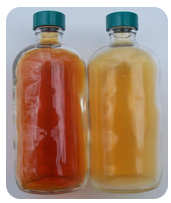# 18.12: Reaction Intermediate

Ozone $$\left( \ce{O_3} \right)$$ depletion in the atmosphere is of significant concern. This gas serves as a protection against the ultraviolet rays of the sun. Ozone is naturally depleted in addition to the depletion caused by human-made chemicals. The depletion reaction is a two-step process:

$\ce{O_3} + \text{ultraviolet light} \rightarrow \ce{O_2} + \ce{O} \cdot \: \text{(free radical) slow reaction}$

$\ce{O} \cdot + \ce{O_3} \rightarrow 2 \ce{O_2} \: \text{fast reaction}$

The free radical is not a part of the overall equation, but can be detected in the lab.

## Intermediate

Reaction mechanisms describe how the material in a chemical reaction gets from the initial reactants to the final products. One reaction that illustrates a reaction mechanism is the reaction between nitrogen monoxide and oxygen to form nitrogen dioxide:

$2 \ce{NO} \left( g \right) + \ce{O_2} \left( g \right) \rightarrow 2 \ce{NO_2} \left( g \right)$

It may seem as though this reaction would occur as the result of a collision between two $$\ce{NO}$$ molecules with one $$\ce{O_2}$$ molecule. However, careful analysis of the reaction has detected the presence of $$\ce{N_2O_2}$$ during the reaction. A proposed mechanism for the reaction consists of two elementary steps:

Step 1: $$2 \ce{NO} \left( g \right) \rightarrow \ce{N_2O_2} \left( g \right)$$

Step 2: $$\ce{N_2O_2} \left( g \right) + \ce{O_2} \left( g \right) \rightarrow 2 \ce{NO_2} \left( g \right)$$

In the first step, two molecules of $$\ce{NO}$$ collide to form a molecule of $$\ce{N_2O_2}$$. In the second step, that molecule of $$\ce{N_2O_2}$$ collides with a molecule of $$\ce{O_2}$$ to produce two molecules of $$\ce{NO_2}$$. The overall chemical reaction is the sum of the two elementary steps:

\begin{align} 2 \ce{NO} \left( g \right) &\rightarrow \cancel{\ce{N_2O_2} \left( g \right)} \\ \cancel{\ce{N_2O_2} \left( g \right)} + \ce{O_2} \left( g \right) &\rightarrow 2 \ce{NO_2} \left( g \right) \\ \hline 2 \ce{NO} \left( g \right) + \ce{O_2} \left( g \right) &\rightarrow 2 \ce{NO_2} \left( g \right) \end{align}

The $$\ce{N_2O_2}$$ molecule is not part of the overall reaction. It was produced in the first elementary step, then reacts in the second elementary step. An intermediate is a species which appears in the mechanism of a reaction, but not in the overall balanced equation. An intermediate is always formed in an early step in the mechanism and consumed in a later step.Figure 18.12.1: Nitrogen dioxide (left) and dinitrogen tetroxide (right). (CC BY-NC; CK-12)

## Summary

• The role of intermediates in reaction mechanisms is described.

## Contributors

• CK-12 Foundation by Sharon Bewick, Richard Parsons, Therese Forsythe, Shonna Robinson, and Jean Dupon.